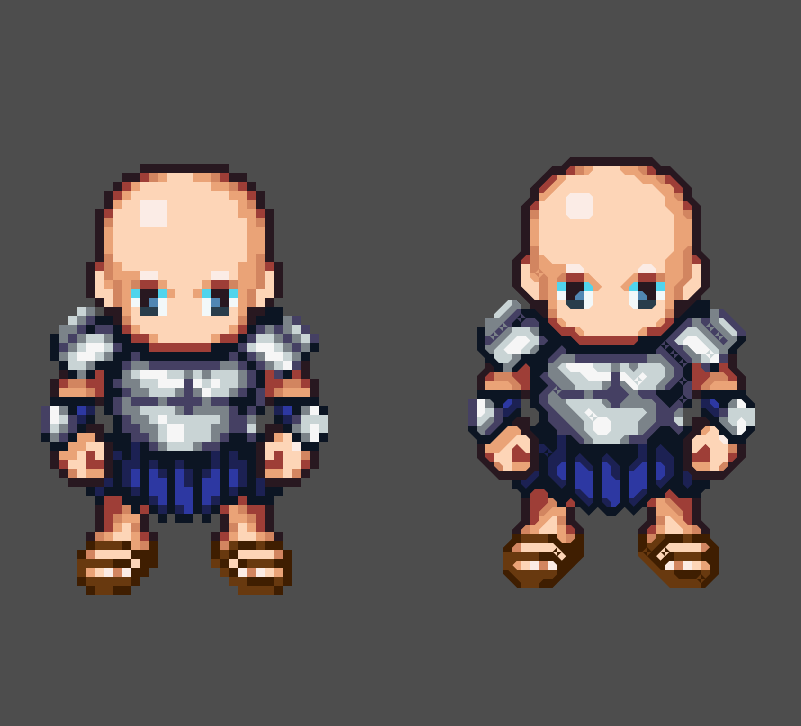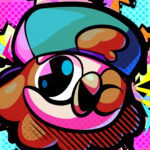# Basic Vector Sprite Upscaling

This is basically a simpler version of the HQ4X shader I posted: NekotoArts HQ4X Shader

Instead of using HQX it just uses a vector between similar pixels and draws a line.

This can be cleaner than the HQ4X Shader and less muddy in some cases.

Still though, I’m not sure how useful this is.

``````shader_type canvas_item;

/*
Made with a whole lot of nightmare fuel.
Logic's Under Pressure and Bobby Tarantino II Albums was in rotation during
the making process.
Hope you enjoy!

You're auto-friended to me if you're a Logic fan
RattPack all day!
*/

//upscaling multiplier amount
const float SCALE = 10.0;

//image mipmap level, for base upscaling
const int ML = 0;

//equality threshold of 2 colors before forming lines
uniform float THRESHOLD = 0.1;

//anti aliasing scaling, smaller value make lines more blurry
uniform float AA_SCALE = 2.0;

//draw diagonal line connecting 2 pixels if within threshold
vec4 diag(vec4 sum, vec2 uv, vec2 p1, vec2 p2, sampler2D iChannel0, float LINE_THICKNESS) {
vec4 v1 = texelFetch(iChannel0,ivec2(uv+vec2(p1.x,p1.y)),ML),
v2 = texelFetch(iChannel0,ivec2(uv+vec2(p2.x,p2.y)),ML);
if (length(v1-v2) < THRESHOLD) {
vec2 dir = p2-p1,
lp = uv-(floor(uv+p1)+.5);
dir = normalize(vec2(dir.y,-dir.x));
float l = clamp((LINE_THICKNESS-dot(lp,dir))*AA_SCALE,0.,1.);
sum = mix(sum,v1,l);
}
return sum;
}

void fragment()
{
float LINE_THICKNESS = 0.4;
vec2 ip = UV;
ip = UV * (1.0 / TEXTURE_PIXEL_SIZE);

vec4 s = texelFetch(TEXTURE,ivec2(ip),ML);

//draw anti aliased diagonal lines of surrounding pixels as 'foreground'
s = diag(s,ip,vec2(-1,0),vec2(0,1), TEXTURE, LINE_THICKNESS);
s = diag(s,ip,vec2(0,1),vec2(1,0), TEXTURE, LINE_THICKNESS);
s = diag(s,ip,vec2(1,0),vec2(0,-1), TEXTURE, LINE_THICKNESS);
s = diag(s,ip,vec2(0,-1),vec2(-1,0), TEXTURE, LINE_THICKNESS);

COLOR = s;
}``````
###### Tags
2d, sprites, upscale, upscalingThe shader code and all code snippets in this post are under CC0 license and can be used freely without the author's permission. Images and videos, and assets depicted in those, do not fall under this license. For more info, see our License terms.

## More from NekotoArts

### 2D Cel / Toon Shader v2 (Basic)

Subscribe
Notify ofInline FeedbacksYodaMax
2 years ago

Would be awesome to make this a screen filter (upscale the whole screen and not just the sprite)JAs
1 year ago

I second this request please! How can we make this into a screen based texture?Firerabbit
7 months ago

The number of texture samples can be reduced from 9 to 5 by sampling outside of the “diag” function.

```shader_type canvas_item;

/*
Made with a whole lot of nightmare fuel.
Logic's Under Pressure and Bobby Tarantino II Albums was in rotation during
the making process.
Hope you enjoy!

You're auto-friended to me if you're a Logic fan
RattPack all day!
*/

//upscaling multiplier amount
const float SCALE = 10.0;

//image mipmap level, for base upscaling
const int ML = 0;

//equality threshold of 2 colors before forming lines
uniform float THRESHOLD = 0.001;

//anti aliasing scaling, smaller value make lines more blurry
uniform float AA_SCALE = 11.0;

//draw diagonal line connecting 2 pixels if within threshold
vec4 diag(vec4 sum, vec2 uv, vec2 p1, vec2 p2, vec4 c1, vec4 c2, float LINE_THICKNESS) {
if (length(c1 - c2) < THRESHOLD) {
vec2 dir = p2-p1,
lp = uv-(floor(uv+p1)+.5);
dir = normalize(vec2(dir.y,-dir.x));
float l = clamp((LINE_THICKNESS-dot(lp,dir))*AA_SCALE,0.,1.);
sum = mix(sum,c1,l);
}
return sum;
}

void fragment()
{
float LINE_THICKNESS = 0.4;
vec2 ip = UV;
ip = UV * (1.0 / TEXTURE_PIXEL_SIZE);

vec4 texCenter = texelFetch(TEXTURE,ivec2(ip),ML);
vec4 texLeft = texelFetch(TEXTURE,ivec2(ip+vec2(-1,0)),ML);
vec4 texUp = texelFetch(TEXTURE,ivec2(ip+vec2(0,1)),ML);
vec4 texRight = texelFetch(TEXTURE,ivec2(ip+vec2(1,0)),ML);
vec4 texDown = texelFetch(TEXTURE,ivec2(ip+vec2(0,-1)),ML);

vec4 s = texCenter;

//draw anti aliased diagonal lines of surrounding pixels as 'foreground'
s = diag(s,ip,vec2(-1,0),vec2(0,1), texLeft, texUp, LINE_THICKNESS);
s = diag(s,ip,vec2(0,1),vec2(1,0), texUp, texRight, LINE_THICKNESS);
s = diag(s,ip,vec2(1,0),vec2(0,-1), texRight, texDown, LINE_THICKNESS);
s = diag(s,ip,vec2(0,-1),vec2(-1,0), texDown, texLeft, LINE_THICKNESS);

COLOR = s;
}
```6 months ago

I really love the look this creates! But does anyone know how to get rid of those weird diamond artifacts like the ones you see in the example dude’s sandals?